xPiezoresistive effectEncyclopedia
The piezoresistive effect describe the changing resistivity
Resistivity
Electrical resistivity is a measure of how strongly a material opposes the flow of electric current. A low resistivity indicates a material that readily allows the movement of electric charge. The SI unit of electrical resistivity is the ohm metre...

of a semiconductor
Semiconductor
A semiconductor is a material with electrical conductivity due to electron flow intermediate in magnitude between that of a conductor and an insulator. This means a conductivity roughly in the range of 103 to 10−8 siemens per centimeter...

due to applied mechanical stress. The piezoresistive effect differs from the piezoelectric effect. In contrast to the piezoelectric effect, the piezoresistive effect only causes a change in electrical resistance
Electrical resistance
The electrical resistance of an electrical element is the opposition to the passage of an electric current through that element; the inverse quantity is electrical conductance, the ease at which an electric current passes. Electrical resistance shares some conceptual parallels with the mechanical...

; it does not produce an electric potential.

## History

The change of resistance in metal devices due to an applied mechanical load was first discovered in 1856 by Lord Kelvin.
With single crystal silicon
Silicon
Silicon is a chemical element with the symbol Si and atomic number 14. A tetravalent metalloid, it is less reactive than its chemical analog carbon, the nonmetal directly above it in the periodic table, but more reactive than germanium, the metalloid directly below it in the table...

becoming the material of choice for the design of analog and digital circuit
Digital circuit
Digital electronics represent signals by discrete bands of analog levels, rather than by a continuous range. All levels within a band represent the same signal state...

s, the large piezoresistive effect in silicon and germanium was first discovered in 1954 (Smith 1954).

## Mechanism

In semiconductors, changes in inter-atomic spacing resulting from strain affects the bandgaps making it easier (or harder depending on the material and strain) for electrons to be raised into the conduction band
Conduction band
In the solid-state physics field of semiconductors and insulators, the conduction band is the range of electron energies, higher than that of the valence band, sufficient to free an electron from binding with its individual atom and allow it to move freely within the atomic lattice of the material...

. This results in a change in resistivity of the semiconductor. Piezoresistivity is defined by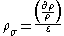Where
∂ρ = Change in resistivity
ρ = Original resistivity
ε = Strains

Piezoresistivity has a much greater effect on resistance than a simple change in geometry and so a semiconductor can be used to create a much more sensitive strain gauge
Strain gauge
A strain gauge is a device used to measure the strain of an object. Invented by Edward E. Simmons and Arthur C. Ruge in 1938, the most common type of strain gauge consists of an insulating flexible backing which supports a metallic foil pattern. The gauge is attached to the object by a suitable...

, though they are generally also more sensitive to environmental conditions (esp. temperature).

### Resistance change in metals

The resistance change in metals is only due to the change of geometry resulting from applied mechanical stress and can be calculated using the simple resistance equation derived from ohm's law
Ohm's law
Ohm's law states that the current through a conductor between two points is directly proportional to the potential difference across the two points...

;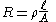where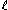Conductor length [m]
A Cross-sectional area of the current flow [m²]

### Piezoresistive effect in semiconductors

The piezoresistive effect of semiconductor materials can be several orders of magnitudes larger than the geometrical effect in metals and is present in materials like germanium
Germanium
Germanium is a chemical element with the symbol Ge and atomic number 32. It is a lustrous, hard, grayish-white metalloid in the carbon group, chemically similar to its group neighbors tin and silicon. The isolated element is a semiconductor, with an appearance most similar to elemental silicon....

, polycrystalline silicon, amorphous silicon, silicon carbide, and single crystal silicon.

#### Piezoresistive effect in silicon

The resistance of silicon changes not only due to the stress dependent change of geometry, but also due to the stress dependent resistivity of the material. This results in gauge factor
Gauge factor
Gauge factor or strain factor of a strain gauge is the ratio of relative change in electrical resistance to the mechanical strain ε, which is the relative change in length.In practice, the resistance is also dependent on temperature...

s to orders of magnitudes larger than those observed in metals (Smith 1954). The resistance of n-conducting
N-type semiconductor
N-type semiconductors are a type of extrinsic semiconductor where the dopant atoms are capable of providing extra conduction electrons to the host material . This creates an excess of negative electron charge carriers....

silicon mainly changes due to a shift of the three different conducting valley pairs. The shifting causes a redistribution of the carriers between valleys with different mobilities. This results in varying mobilities dependent on the direction of current flow. A minor effect is due to the effective mass change related to changing shapes of the valleys. In p-conducting silicon the phenomena are more complex and also result in mass changes and hole transfer.

## Piezoresistive silicon devices

The piezoresistive effect of semiconductors has been used for sensor devices employing all kinds of semiconductor materials such as germanium
Germanium
Germanium is a chemical element with the symbol Ge and atomic number 32. It is a lustrous, hard, grayish-white metalloid in the carbon group, chemically similar to its group neighbors tin and silicon. The isolated element is a semiconductor, with an appearance most similar to elemental silicon....

, polycrystalline silicon, amorphous silicon, and single crystal silicon. Since silicon is today the material of choice for integrated digital and analog circuits the use of piezoresistive silicon devices has been of great interest. It enables the easy integration of stress sensors with Bipolar and CMOS circuits.

This has enabled a wide range of products using the piezoresistive effect. Many commercial devices such as pressure sensors and acceleration
Acceleration
In physics, acceleration is the rate of change of velocity with time. In one dimension, acceleration is the rate at which something speeds up or slows down. However, since velocity is a vector, acceleration describes the rate of change of both the magnitude and the direction of velocity. ...

sensors employ the piezoresistive effect in silicon
Silicon
Silicon is a chemical element with the symbol Si and atomic number 14. A tetravalent metalloid, it is less reactive than its chemical analog carbon, the nonmetal directly above it in the periodic table, but more reactive than germanium, the metalloid directly below it in the table...

. But due to its magnitude the piezoresistive effect in silicon has also attracted the attention of research and development for all other devices using single crystal silicon. Semiconductor
Semiconductor
A semiconductor is a material with electrical conductivity due to electron flow intermediate in magnitude between that of a conductor and an insulator. This means a conductivity roughly in the range of 103 to 10−8 siemens per centimeter...

Hall sensors, for example, were capable of achieving their current precision only after employing methods which eliminate signal contributions due the applied mechanical stress.

### Piezoresistors

Piezoresistors are resistors made from a piezoresistive material and are usually used for measurement of mechanical
stress
Stress (physics)
In continuum mechanics, stress is a measure of the internal forces acting within a deformable body. Quantitatively, it is a measure of the average force per unit area of a surface within the body on which internal forces act. These internal forces are a reaction to external forces applied on the body...

. They are the simplest form of piezoresistive devices.

#### Fabrication

Piezoresistors can be fabricated using wide variety of piezoresistive materials. The simplest form of piezoresistive silicon sensors are diffused resistors. Piezoresistors consist of a simple two contact diffused n- or p-wells within a p- or n-substrate. As the typical square resistances of these devices are in the range of several hundred ohms, additional p+ or n+ plus diffusions are necessary to facilitate ohmic contacts to the device.
Schematic cross-section of the basic elements of a silicon n-well piezoresistor.

#### Physics of operation

For typical stress values in the MPa
Pascal (unit)
The pascal is the SI derived unit of pressure, internal pressure, stress, Young's modulus and tensile strength, named after the French mathematician, physicist, inventor, writer, and philosopher Blaise Pascal. It is a measure of force per unit area, defined as one newton per square metre...

range the stress dependent voltage drop along the resistor Vr, can be considered to be linear. A piezoresistor aligned with the x-axis as shown in the figure may be described by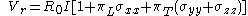where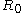, I,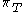,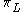, and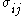denote the stress free resistance, the applied current, the transverse and longitudinal piezoresistive coefficients, and the three tensile stress components, respectively. The piezoresistive coefficients vary significantly with the sensor orientation with respect to the crystallographic axes and with the doping profile. Despite the fairly large stress sensitivity of simple resistors, they are preferably used in more complex configurations eliminating certain cross sensitivities and drawbacks. Piezoresistors have the disadvantage of being highly sensitive to temperature changes while featuring comparatively small relative stress dependent signal amplitude changes.
Advanced stress sensors derived from piezoresistors are Wheatstone bridge
Wheatstone bridge
A Wheatstone bridge is an electrical circuit used to measure an unknown electrical resistance by balancing two legs of a bridge circuit, one leg of which includes the unknown component. Its operation is similar to the original potentiometer. It was invented by Samuel Hunter Christie in 1833 and...

s, transducers, and picture frame sensors.

### Other piezoresistive devices

In silicon the piezoresistive effect is used in piezoresistors, transducers, piezo-FETS, solid state accelerometers and bipolar transistors.